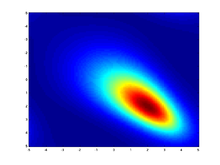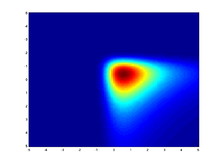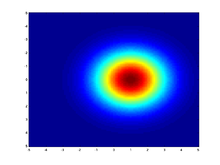# Multivariate stable distribution

Multivariate stable distribution
parameters: Probability density functionHeatmap showing a Multivariate (bivariate) stable distribution with α = 1.1$\alpha \in (0,2]$ — exponent$\delta \in \mathbb{R}^d$ - shift/location vector Λ(s) - a spectral finite measure on the sphere$u \in \mathbb{R}^d$ (no analytic expression) (no analytic expression) Infinite when α < 2 see text

The multivariate stable distribution is a multivariate probability distribution that is a multivariate generalisation of the univariate stable distribution. The multivariate stable distribution defines linear relations between stable distribution marginals.[clarification needed] In the same way as for the univariate case, the distribution is defined in terms of its characteristic function.

The multivariate stable distribution can also be thought as an extension of the multivariate normal distribution. It has parameter, α, which is defined over the range 0 < α ≤ 2, and where the case α = 2 is equivalent to the multivariate normal distribution. It has an additional skew parameter that allows for non-symmetric distributions, where the multivariate normal distribution is symmetric.

## Definition

Let S be the unit sphere in$R^d : \mathbb{S} = \{u \in R^d : |u| = 1\}$. For a random variable, X, it has a multivariate stable distribution and the notation XS(α,Λ,δ) is used, if the joint characteristic function of X is$\operatorname{E} \exp(u^T X) = \exp \left\{-\int \limits_{S \in R^d}\left\{|u^Ts|^\alpha + i \nu (u^Ts, \alpha) \right\} \, \Lambda(ds) + u^T\delta\right\}$

where 0 < α < 2, and$\nu(u,\alpha) =\begin{cases} -\mathbf{sign}(u) \tan(\pi \alpha / 2)|u|^\alpha & \alpha \ne 1, \\ (2/\pi)u \ln |u| & \alpha=1. \end{cases}$

This is essentially the result of Feldheim, that any stable random vector can be characterized by a spectral measure Λ (a finite measure on S) and a shift vector$\delta \in R^d$.

## Parametrization using projections

Another way to describe a stable random vector is in terms of projections. For any vector u, the projection uTX is univariate α − stable with some skewness β(u), scale γ(u) and some shift δ(u). The notation$X \sim S(\alpha,\beta(\cdot),\gamma(\cdot),\delta(\cdot))$ is used if uTX is stable with$u^TX \sim s(\alpha,\beta(\cdot),\gamma(\cdot),\delta(\cdot))$ for every$u \in R^d$. This is called the projection parameterization.

The spectral measure determines the projection parameter functions by:$\gamma(u) = \int_{s \in \mathbb{S}} |u^Ts|^\alpha \Lambda(ds)$$\beta(u) = \int_{s \in \mathbb{S}}|u^Ts|^\alpha \mathbf{sign}(u^Ts)\Lambda(ds)$$\delta(u)=\begin{cases}u^T \delta & \alpha \ne 1\\u^T \delta -\int_{s \in \mathbb{S}}\tfrac{\pi}{2} u^Ts \ln|u^Ts|\Lambda(ds)&\alpha=1\end{cases}$

## Special cases

There are four special cases where the multivariate characteristic function takes a simpler form. Define the characteristic function of a stable marginal as$\omega(u|\alpha,\beta) = \begin{cases}|u|^\alpha\left[1-i \beta(\tan \tfrac{\pi\alpha}{2})\mathbf{sign}(u)\right]& \alpha \ne 1\\ |u|\left[1+i \beta \tfrac{2}{\pi} \mathbf{sign}(u)\ln |u|\right] & \alpha = 1\end{cases}$

### Isotropic multivariate stable distribution

The characteristic function is$E \exp(i u^T X)=\exp\{-\gamma_0^\alpha+i u^T \delta)\}$ The spectral measure is continuous and uniform, leading to radial/isotropic symmetry.

### Elliptically contoured multivariate stable distribution

Elliptically contoured m.v. stable distribution is a special symmetric case of the multivariate stable distribution. If X is α-stable and elliptically contoured, then it has joint characteristic function Eexp(iuTX) = exp{ − (uTΣu)α / 2 + iuTδ)} for some positive definite matrix Σ and shift vector$\delta \in R^d$. Note the relation to characteristic function of the multivariate normal distribution: Eexp(iuTX) = exp{ − (uTΣu) + iuTδ)}. In other words, when α = 2 we get the characteristic function of the multivariate normal distribution.

### Independent components

The marginals are independent with XjS(α,βjjj), then the characteristic function is$E \exp(i u^T X) = \exp\left\{-\sum_{j=1}^m \omega(u_j|\alpha,\beta_j)\gamma_j^\alpha +i u^T \delta)\right\}$Heatmap showing a multivariate (bivariate) independent stable distribution with α = 1Heatmap showing a multivariate (bivariate) independent stable distribution with α = 2.

### Discrete

If the spectral measure is discrete with mass λj at$s_j \in \mathbb{S},j=1,\ldots,m$ the characteristic function is$E \exp(i u^T X)= \exp\left\{-\sum_{j=1}^m \omega(u^Ts_j|\alpha,1)\gamma_j^\alpha +i u^T \delta)\right\}$

## Linear properties

if$X \sim S(\alpha, \beta(\cdot), \gamma(\cdot), \delta(\cdot))$ is d-dim, and A is a m x d matrix,$b \in \mathbb{R}^m$ then AX + b is m dim. α-stable with scale function$\gamma(A^T\cdot)$ , skewness function$\beta(A^T\cdot)$, and location function$\delta(A^T\cdot) + b^T\cdot$

## Inference in the independent component model

Recently it was shown how to compute inference in closed-form in a linear model (or equivalently a factor analysis model),involving independent component models.

More specifically, let$X_i \sim S(\alpha, \beta_{x_i}, \gamma_{x_i}, \delta_{x_i}), i=1,\ldots,n$ be a set of i.i.d. unobserved univariate drawn from a stable distribution. Given a known linear relation matrix A of size$n \times n$, the observation$Y_i = \sum_{i=1}^n A_{ij}X_j$ are assumed to be distributed as a convolution of the hidden factors Xi.$Y_i = S(\alpha, \beta_{y_i}, \gamma_{y_i}, \delta_{y_i})$. The inference task is to compute the most probable Xi, given the linear relation matrix A and the observations Yi. This task can be computed in closed-form in O(n3).

An application for this construction is multiuser detection with stable, non-Gaussian noise.

## Resources

Wikimedia Foundation. 2010.

Поможем написать реферат

### Look at other dictionaries:

• Multivariate normal distribution — MVN redirects here. For the airport with that IATA code, see Mount Vernon Airport. Probability density function Many samples from a multivariate (bivariate) Gaussian distribution centered at (1,3) with a standard deviation of 3 in roughly the… …   Wikipedia

• Multivariate Student distribution — Multivariate Student parameters: location (real vector) Σ scale matrix (positive definite real matrix) n is the degree of freedom support …   Wikipedia

• Multivariate Pólya distribution — The multivariate Pólya distribution, named after George Pólya, also called the Dirichlet compound multinomial distribution, is a compound probability distribution, where a probability vector p is drawn from a Dirichlet distribution with parameter …   Wikipedia

• Geometric stable distribution — Geometric Stable parameters: α ∈ (0,2] stability parameter β ∈ [−1,1] skewness parameter (note that skewness is undefined) λ ∈ (0, ∞) scale parameter μ ∈ (−∞, ∞) location parameter support: x ∈ R, or x ∈ [μ, +∞) if α < 1 and β = 1, or x ∈… …   Wikipedia

• Joint probability distribution — In the study of probability, given two random variables X and Y that are defined on the same probability space, the joint distribution for X and Y defines the probability of events defined in terms of both X and Y. In the case of only two random… …   Wikipedia

• Multivariate kernel density estimation — Kernel density estimation is a nonparametric technique for density estimation i.e., estimation of probability density functions, which is one of the fundamental questions in statistics. It can be viewed as a generalisation of histogram density… …   Wikipedia

• Cauchy distribution — Not to be confused with Lorenz curve. Cauchy–Lorentz Probability density function The purple curve is the standard Cauchy distribution Cumulative distribution function …   Wikipedia

• Normal distribution — This article is about the univariate normal distribution. For normally distributed vectors, see Multivariate normal distribution. Probability density function The red line is the standard normal distribution Cumulative distribution function …   Wikipedia

• Hypergeometric distribution — Hypergeometric parameters: support: pmf …   Wikipedia

• Dirichlet distribution — Several images of the probability density of the Dirichlet distribution when K=3 for various parameter vectors α. Clockwise from top left: α=(6, 2, 2), (3, 7, 5), (6, 2, 6), (2, 3, 4). In probability and… …   Wikipedia